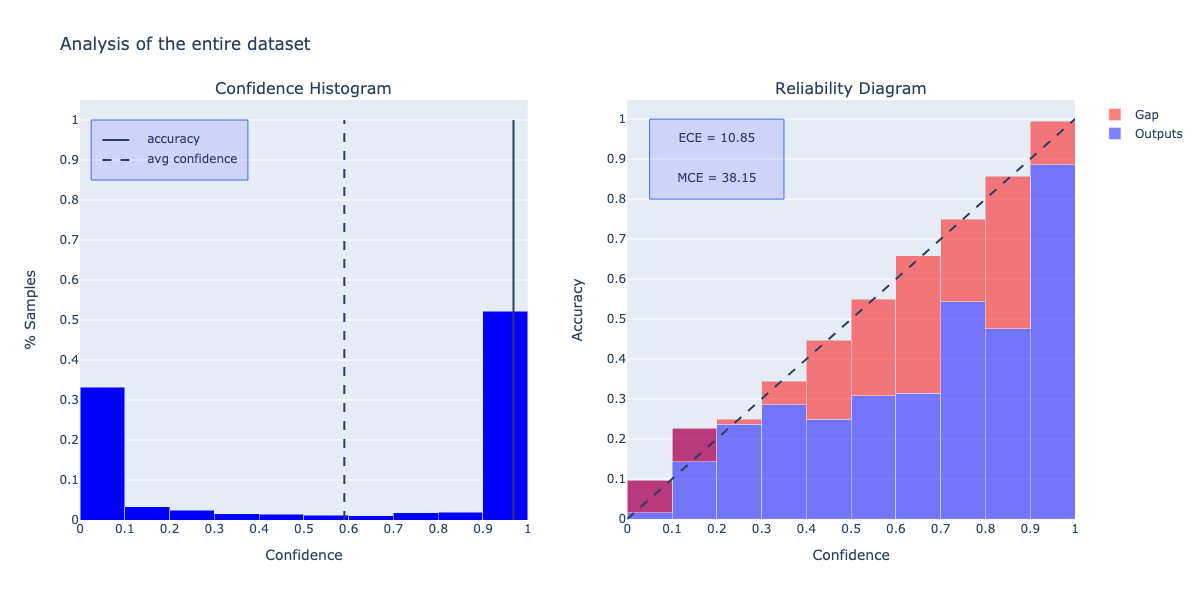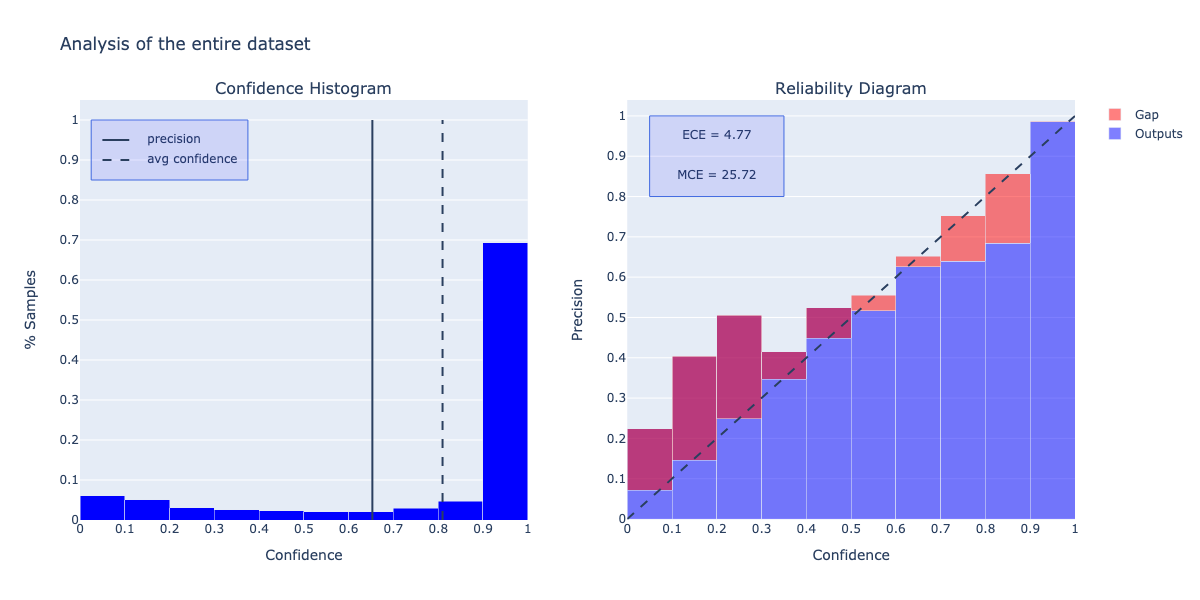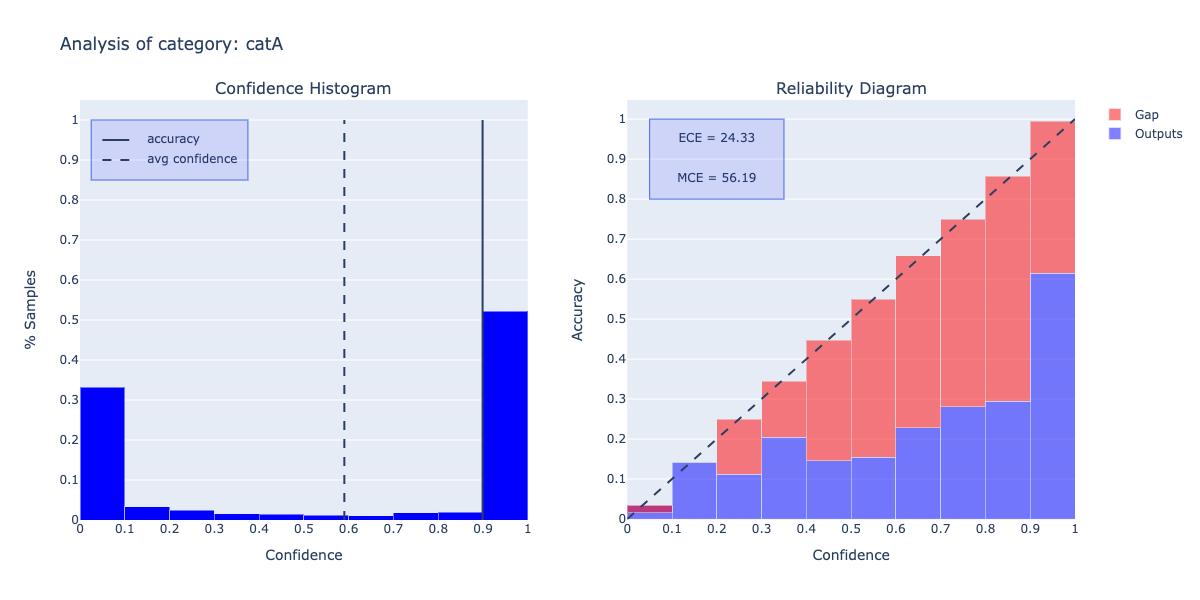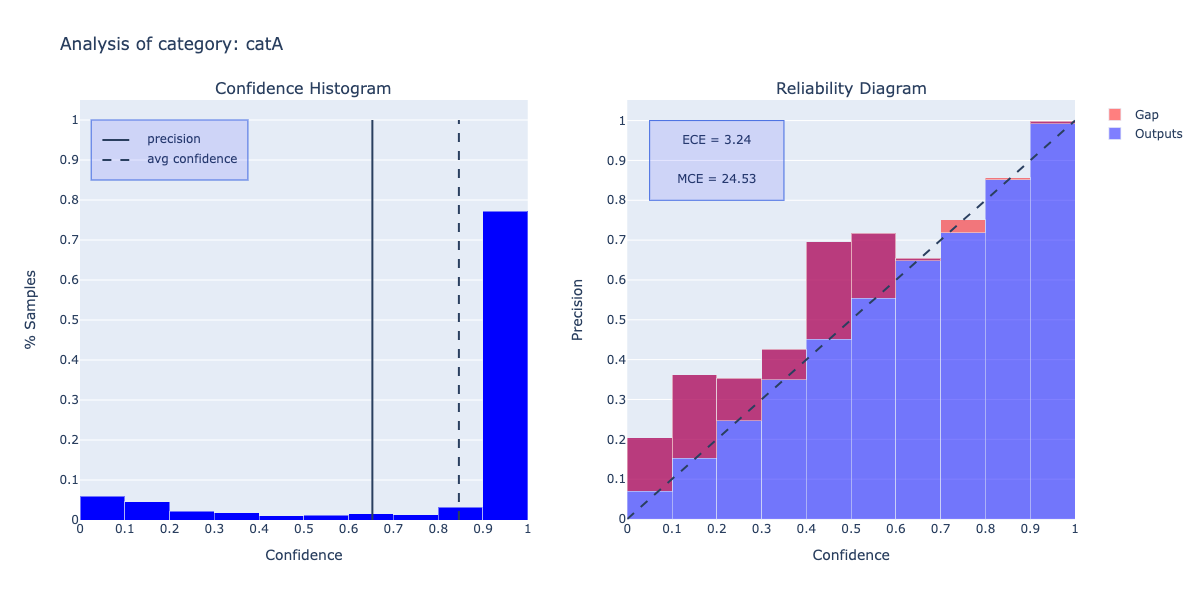# analyze_reliability()

It provides the reliability analysis by showing the distribution of the proposals among different confidence values and the corresponding confidence calibration.

#### Parameters

bins

int, optional
The number of bins the confidence values are split into.
(default is 10)
show

bool, optional
Indicates whether the plot should be shown or not. If False, returns the results as dict.
(default is True)

### Example

#### Classification

``````from odin.classes import AnalyzerClassification

my_analyzer = AnalyzerClassification("my_classifier_name", my_classification_dataset)
my_analyzer.analyze_reliability()
``````#### Localization

``````from odin.classes import AnalyzerLocalization

my_analyzer = AnalyzerLocalization("my_detector_name", my_localization_dataset)
my_analyzer.analyze_reliability()
``````Binary Classification Single-label Classification Multi-label Classification Object Detection Instance Segmentation
yes yes yes yes yes

# analyze_reliability_for_categories()

It provides the reliability analysis by showing the distribution of the proposals among different confidence values and the corresponding confidence calibration for each category.

#### Parameters

categories

list, optional
If not specified, it performs the analysis on the entire data set, otherwise it performs a per-category analysis.
(default is None)
bins

int, optional
The number of bins the confidence values are split into.
(default is 10)
show

bool, optional
Indicates whether the plot should be shown or not. If False, returns the results as dict.
(default is True)

### Example

#### Classification

``````from odin.classes import AnalyzerClassification

my_analyzer = AnalyzerClassification("my_classifier_name", my_classification_dataset)
my_analyzer.analyze_reliability_for_categories()
``````N.B. As example, it is shown only the output of a single category, but the analysis is performed for all the categories selected.

#### Localization

``````from odin.classes import AnalyzerLocalization

my_analyzer = AnalyzerLocalization("my_detector_name", my_localization_dataset)
my_analyzer.analyze_reliability_for_categories()
``````N.B. As example, it is shown only the output of a single category, but the analysis is performed for all the categories selected.